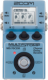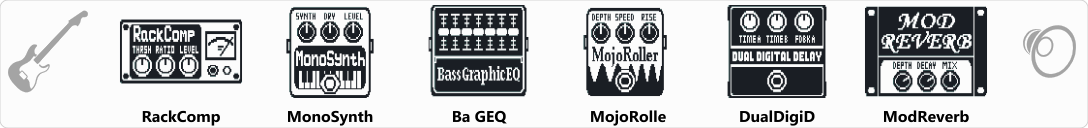# Theremin EBOW

Discussion in 'Zoom MS-70CDR' started by Israel cezar, Jan 26, 2021.

1. Theremin EBOWDevice: Zoom MS-70cdr
Firmware: 2.10

Name on device: Theremin
Optimized for: Guitar Amp

Effects chain:As if it were a EBOW

Effect: "RackComp" (Dynamics / Filter), active - "yes"
"THRSH" = 45
"Ratio" = 5
"Level" = 100
"ATTCK" = 4

Effect: "MonoSynth" (Modulation / Sfx), active - "yes"
"Synth" = 70
"Dry" = 0
"Level" = 100
"Wave" = Sine
"Tone" = 10
"Speed" = 100

Effect: "Ba GEQ" (Dynamics / Filter), active - "yes"
"50Hz" = -7
"120Hz" = -6
"400Hz" = -1
"500Hz" = -10
"800Hz" = 5
"4.5kHz" = 5
"10kHz" = 5
"Level" = 100

Effect: "MojoRolle" (Modulation / Sfx), active - "yes"
"Depth" = 25
"Speed" = 55
"Rise" = 43
"Mode" = Up-Dn
"Level" = 100

Effect: "DualDigiD" (Delay / Reverb), active - "yes"
"TimeA" = 397
"TimeB" = 375
"FdbkA" = 50
"FdbkB" = 50
"Depth" = 101
"Speed" = 25
"FLTR" = -50
"DlyMx" = 25
"Mix" = 50

Effect: "ModReverb" (Delay / Reverb), active - "yes"
"Depth" = 80
"Decay" = 15
"Mix" = 60
"Rate" = 10
"Tone" = 10
"PreD" = 3
"Level" = 100
"Tail" = Off

Note: This is a patch file, you will need to download and install the ToneLib-Zoom software to use the patch.

File size:
435 bytes
Views:
334